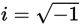# SAT Math Multiple Choice Question 530: Answer and Explanation

### Test Information

Question: 530

5. Which of the following expressions is equivalent to (6 + 5i)3? (Note:)

• A. 11 + 60i
• B. 216 – 125i
• C. –234 + 415i
• D. –3,479 + 1,320i

Explanation:

C

Difficulty: Medium

Category: Additional Topics in Math / Imaginary Numbers

Strategic Advice: You will not be expected to raise a complex number like the one in this question to the third power by hand. That's a clue that you should be able to use your calculator.

Getting to the Answer: The definition of i has been programmed into all graphing calculators, so you can perform basic operations on complex numbers using the calculator (in the Calculator Section of the test). Enter the expression as follows: (6 + 5i)3. On the TI83/84 calculators, you can find i on the button with the decimal point. After entering the expression, the calculator should return –234 + 415i, which is (C).

You could, however, expand the number by hand, by writing it as (6 + 5i)(6 + 5i)(6 + 5i) and carefully multiplying it all out.